# 计算机浮点数规格化表示

### 说明

float

float类型在内存中占用的位数为: 1+8+23=32bits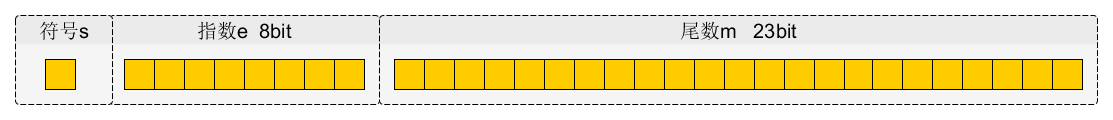double

1+11+52=64bits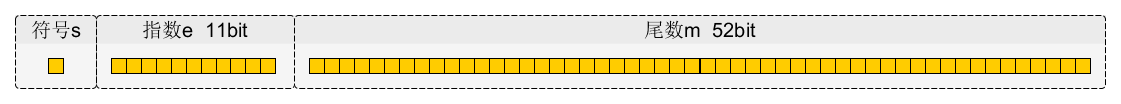1.00001001000101010101000。　用23位表示了24位的信息 (小数点不占位置).

### 表示

x=(−1)^s × (1.M) × 2^E−127

x=(−1)^s × (1.M) × 2^E−1023

(1) 首先转化为2进制表示

−3.75=−(2+1+1/2+1/4)=−1.111×2^1

(2) 整理符号位并进行规格化表示

−1.111×21=(−1)(1)×(1+0.1110 0000 0000 0000 0000 000)×2^1

(3) 进行阶码的移码处理

(−1)^(1)×(1+0.1110 0000 0000 0000 0000 000)×2^1

=(−1)^(1)×(1+0.1110 0000 0000 0000 0000 000)×2^128−127

### 浮点数表示范围与精度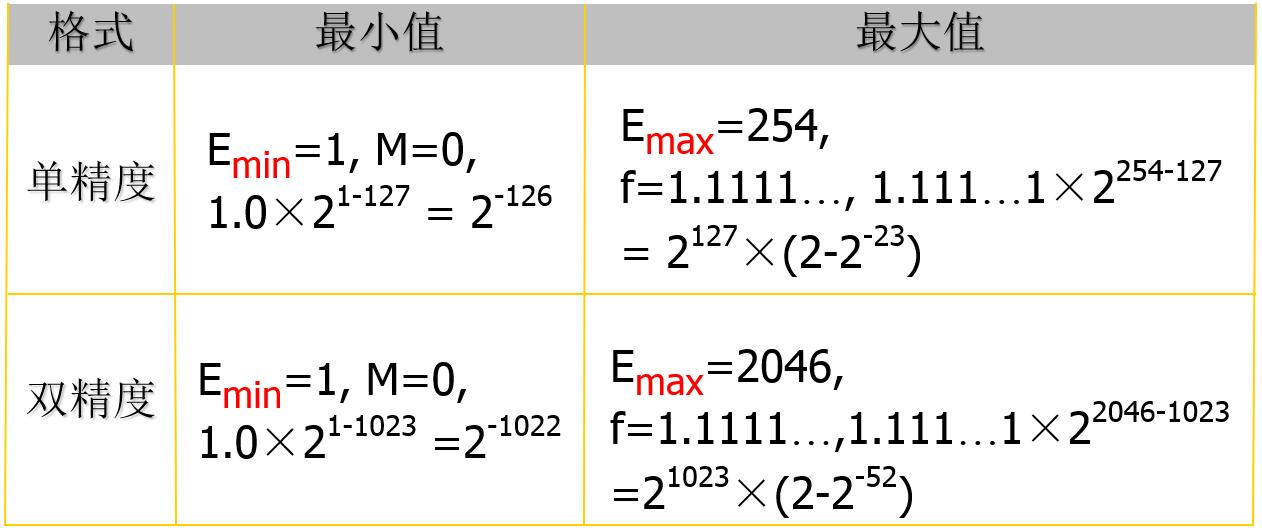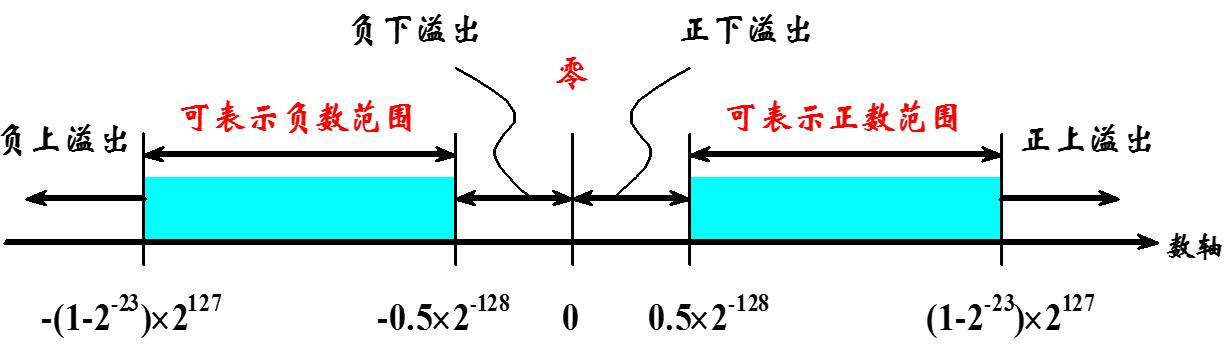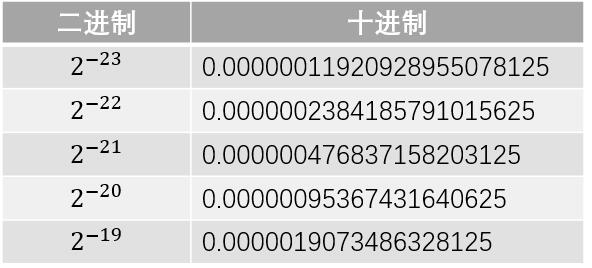2−23 和 2−22 之间是存在间隔的，即0.0000001和0.0000002之间的小数我们是没有办法描述的，因此23位尾数最多只能描述到小数点后第7位；此外，我们通过四舍五入可以很容易发现0.0000003=0.0000004=2−23+2−22, 这表明第7位有效数字只是部分准确。而第6位及之前的都是可以准确描述的，因此我们说float的有效位为6~7位。

### 例子

http://blog.csdn.net/shuzfan/article/details/53814424

http://blog.csdn.net/techx/article/details/43830781

10-16619506-122万+
08-294132
04-201306
01-121460
05-139079
11-084634
12-013796
07-181万+
06-266171